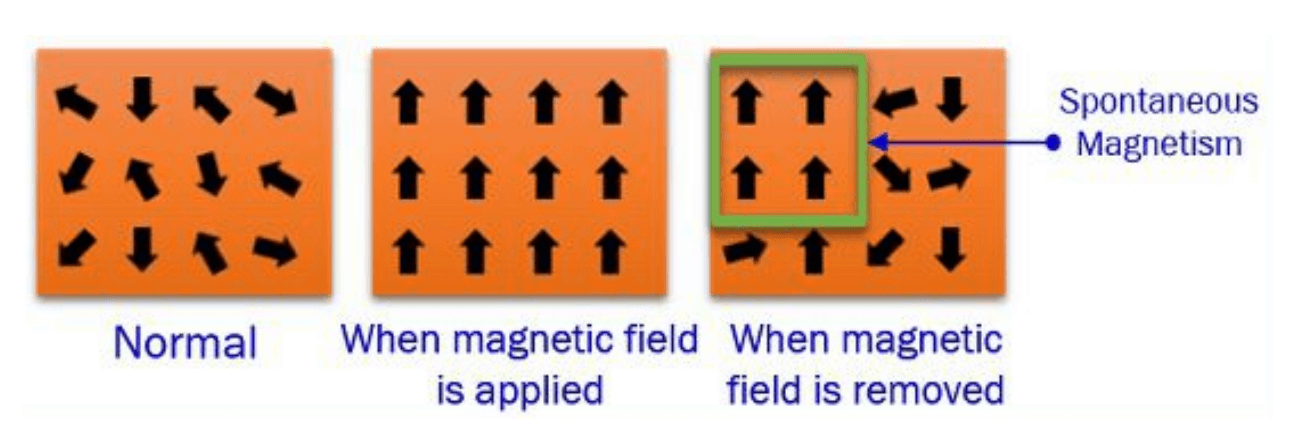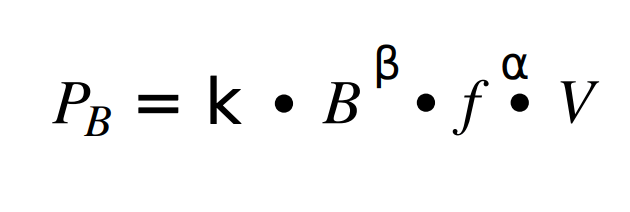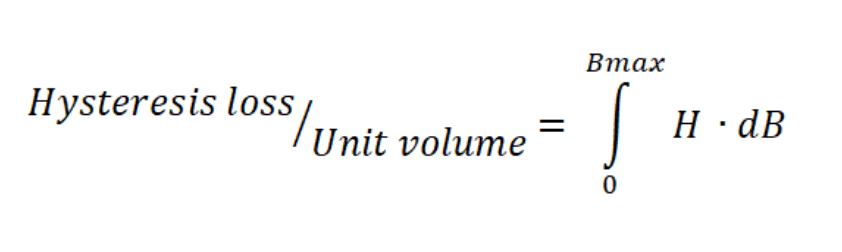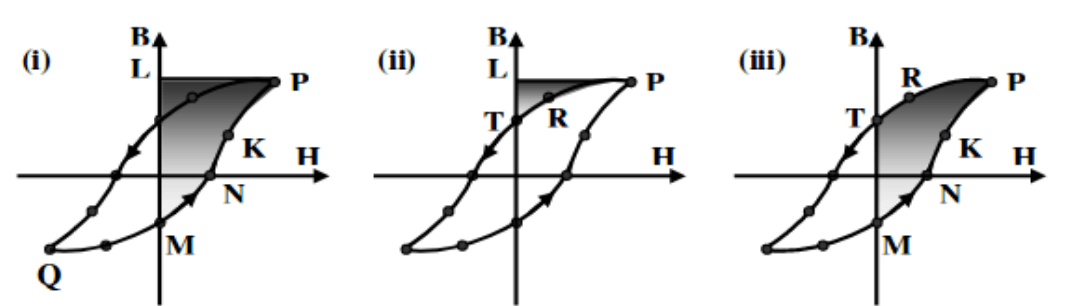# Hysteresis Losses Causes & Calculations

When designing a magnetic component, losses must be taken into account to ensure its correct functionality. In this app note, hysteresis losses will be discussed. As the eddy losses, they are produced in the core due to changes in the magnetic flux density; depend on the material properties and shape, and are released in the form of heat that is undesirable.

### Where do they come from?

Hysteresis losses are caused by the magnetization and demagnetization of a core as current flows in reverse directions and it is released in the form of heat. In fact, as a consequence of the friction of the molecules due to the constant change in module and direction of the magnetic flow lines of the sinusoidal supply voltage, an undesirable increase in temperature is created.Figure 1. Molecular arrangement.

## Hysteresis Loss CalculationWhere:

• PB is the core loss.
• k, β, α are the Steinmetz coefficients, that depend on the core material.
• B is the peak flux density.
• f is the changing frequency.
• V is the volume of the magnetic material. In Frenetic we try to find the perfect magnetic under the specifications given with the lowest volume possible so the hysteresis losses are minimal.

### Hysteresis Loop (or B-H Curve)

The hysteresis loop represents the magnetic flux and magnetization force changes. Its area is proportional to the core losses.The first graph shows the energy absorbed during the sinusoidal excitation when having +H; while in the second, the energy that is returned during this interval. That leaves the area of the third graph as a representation of the total hysteresis losses of the core.Figure 2. Hysteresis loop

Hence, a lower area will mean smaller losses. When selecting a core, the hysteresis loop of each of them should be compared. Hard ferromagnetic materials will have bigger coercive forces (making the loop wider), while soft ferromagnetics will have lower, which makes them more appropriate for transformers.

#### Conclusions

Magnetic cores tend to have narrowed hysteresis loops which will have a lower area (smaller coercive force and remanence) and consequently lower hysteresis losses that will imply a small amount of dissipated energy in the form of heat. These losses not only reduce the efficiency of the magnetic component but also, over time, the excess of heat loss can cause insulating materials life to shorten. Frenetic's artificial intelligence takes into account all different shapes and materials in order to give the customer an optimal design.